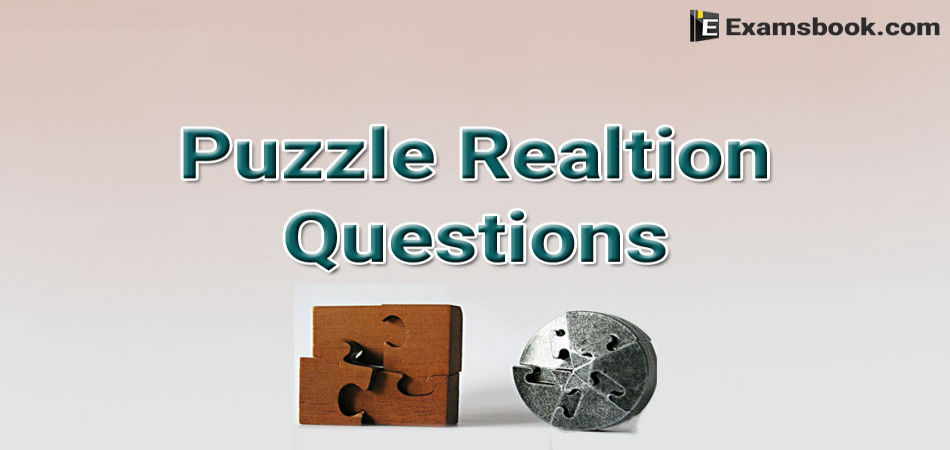• Save

# Puzzle Relation Questions for Competitive Exams

Last year 4.0K ViewsIn puzzle relation questions, a student has to understand the relation to solve a question. These type of questions are not much difficult, its just confusing questions. So, here are the given puzzle relation questions for your practice.

If you understand this topic that how to solve, you will easily improve your score in the competitive exams.

## Important Puzzle Relation Questions

Q.1. A is the son of B.C, Bs sister has a son D and a daughter E.F is the maternal uncle of D. How is E related to F?

(A) Daughter

(B) Sister

(C) Wife

(D) Niece

Ans .   D

E is the daughter of C and D is the son of C. So, F, who is the maternal uncle of D, is also the maternal uncle of E. Thus, E is the niece of F.

Q.2. Rahuls mother is the only daughter of Monikas father. How is Monikas husband related to Rahul?

(A) Father

(B) Uncle

(C) Brother

(D) Grandfather

Ans .   A

Clearly, the only daughter of Monika's father is Monika herself. So, Rahul's mother is Monika.Thus, Monika's husband is the father of Rahul.

Q.3. A is the son of B. C, Bs sister has a son D and a daughter E. F is the maternal uncle of D. How is A related to D?

(A) Nephew

(B) Cousin

(C) Brother

(D) Uncle

Ans .   B

A is the son of B and D is the son of the sister of B.So, A is the cousin of D.

Q.4. If (i) M is brother of N;

(ii) B is brother of N;

(iii) M is brother of D, then which of the following statements is definitely true?

(A) N is brother of D

(B) N is brother of B

(C) D is brother of M

(C) M is brother of B

(E) None of these

Ans .   D

M is brother of B. M is the brother of N and B is the brother of N. So, M is the brother of B.

Q.5. Qs mother is sister of P and daughter of M. S is daughter of P and sister of T. How is M related to T?

(A) Father

(B) Grandmother

(C) Grandfather or Grandmother

(D) Grandfather

(E) None of these

Ans .   B

S is daughter of P and sister of T. So, T is daughter of P. Now, the sister of P is the daughter of M. This means that P is also the daughter of M.  Clearly, T is the granddaughter of M. So, M is the Grandfather or Grandmother of T.

Q.6. Deepak is brother of Ravi. Rekha is sister of Atul. Ravi is son of Rekha. How is Deepak related to Rekha?

(A) Brother

(B) Son

(C) Father

(D) Nephew

Ans .   B

Deepak is the son of Ravi, who is the son of Rekha.Thus, Deepak is the son of Rekha.

Q.7. A is father of C and D is son of B. E is brother of A. If C is sister of D, how is B related to E ?

(A) Brother-in-Law

(B) Daughter

(C) Sister-in-Law

(D) Husband

Ans .   C

A is father of C and C is sister of D. So, A is father of D. But D is son of B. So, B is the mother of D and wife of A. Also, E is the brother of A. So, B is the sister-in-law of E.

Q.8. A is Bs sister. C is Bs mother. D is Cs father. E is Ds mother. Then, how is A related to D?

(A) Grandfather

(B) Grandmother

(C) Grand daughter

(D) Daughter

Ans .   C

A is the sister of B and B is the daughter of C. So, A is the daughter of C. Also, D is the father of C. So, A is the granddaughter of D.

Q.9. Daya has a brother Anil, Daya is the son of Chandra. Bimal is Chandra`s father. In term of relationship, what is Anil of Bimal?

(A) Grandson

(B) Son

(C) Grandfather

(D) Brother

Ans .   A

Anil is the brother of Daya and Daya is the son of Chandra. So, Anil is the son of Chandra. Now, Bimal is the father of Chandra. So, Anil is the grandson of Bimal.

Q.10. Give that:

1. A is brother of B.

2. C is brother of A.

3. D is brother of E.

4. E is brother of B.

Then uncle of D is

(A) B

(B) A

(C) E

(D) C

Ans .   B

Clearly, D is the brother of E and E is the daughter of B. So, D is the son of B. Also, A is the brother of B. So, A is the uncle of D.